# # 如何写一个 2048 小游戏？

## # 一、2048 是什么

2048 是一款滑块类游戏，由意大利程序员 Gabriele Cirulli 编写并在 GitHub 上开源。

「你还写过 2048 ？」

「是啊」

「可以玩吗？」

「不能。。。」

## # 三、开发思路与步骤

### # 3.1 布局

<div id="grid-container">
<div>
<div v-for="(row, rowIndex) in chessBoard" :key="rowIndex">
<div
v-for="(cell, columnIndex) in row"
:key="rowIndex + columnIndex"
class="grid-cell"
:id="'grid-cell-' + rowIndex + '-' + columnIndex"></div>
</div>

<div v-for="(row, rowIndex) in chessBoard" :key="rowIndex">
<div v-for="(cell, columnIndex) in row" :key="columnIndex">
<span
class="number-cell"
:id="'number-cell-' + rowIndex + '-' + columnIndex"
v-show="shouldShowCell(rowIndex, columnIndex)"
v-text="cell"></span>
</div>
</div>
</div>
</div>

1
2
3
4
5
6
7
8
9
10
11
12
13
14
15
16
17
18
19
20
21

### # 3.3 游戏逻辑

#### # 3.3.1 开始游戏

1. 添加键盘事件
document.addEventListener('keyup', processKeyUp);

1

1. 初始化网格

1. 在网格上生成两个随机位置，值是 2 或者 4

• 网格上还存在空间，没有空间就无法生成。
• 随机位置不能是已经有值的位置，我们是生成，不是更新。
• 对随机位置生成的方块添加背景颜色和字体颜色。
/**
* 判断棋盘中还有空间吗
*
* @returns true->还有空间, false->没有
*/
function isChessBoardExistSpace() {
for (let i = 0; i < 4; i++) {
for (let j = 0; j < 4; j++) {
// 还有空间
if (chessBoard[i][j] === 0) {
return false;
}
}
}
return true;
}

/**
* 生成数字
*/
function showNumberWithAnimation(i, j, randNumber) {
let numberCell = document.getElementById('number-cell-' + i + '-' + j);
if (numberCell) {
numberCell.style.top = getPosTop(i, j) + 'px';
numberCell.style.left = getPosLeft(i, j) + 'px';
numberCell.style.width = '100px';
numberCell.style.height = '100px';

// 获取随机数值的背景颜色和字体颜色
numberCell.style.backgroundColor = getNumberBackgroundColor(randNumber);
numberCell.style.color = getNumberColor(randNumber);
numberCell.textContent = randNumber;
}
}

/**
* 随机生成数字
*
* @returns
*/
function generateOneNumber() {
// 棋盘中还有空间就生成数字
if (isChessBoardExistSpace()) {
// 没有空间返回 false
return false;
}

// 随机生成 0-4 的位置不包括 4
let randX = parseInt(Math.floor(Math.random() * 4));
let randY = parseInt(Math.floor(Math.random() * 4));

// 判断位置是否可用
while (true) {
if (chessBoard[randX][randY] === 0) {
// 位置可用跳出死循环，不可用继续找
break;
}
randX = parseInt(Math.floor(Math.random() * 4));
randY = parseInt(Math.floor(Math.random() * 4));
}

// 随机生成 2 或 4，它们的概率相同
var randNumber = Math.random() > 0.5 ? 2 : 4;

// 在随机位置显示随机数字 2 或 4
chessBoard[randX][randY] = randNumber;

showNumberWithAnimation(randX, randY, randNumber);
}

1
2
3
4
5
6
7
8
9
10
11
12
13
14
15
16
17
18
19
20
21
22
23
24
25
26
27
28
29
30
31
32
33
34
35
36
37
38
39
40
41
42
43
44
45
46
47
48
49
50
51
52
53
54
55
56
57
58
59
60
61
62
63
64
65
66
67
68
69

#### # 3.3.2 移动方块

1. 点击上下左右按键
/**
* 点击上下左右按键
*/
function processKeyUp(e) {
var keyCode = e.keyCode;
// 点击上键
if (keyCode === 38) {
if (moveUp()) {
afterMove();
}
return;
}

// 点击左键
if (keyCode === 37) {
if (moveLeft()) {
afterMove();
}
return;
}

if (keyCode === 39) {
if (moveRight()) {
afterMove();
}
return;
}

if (keyCode === 40) {
if (moveDown()) {
afterMove();
}
return;
}
}

1
2
3
4
5
6
7
8
9
10
11
12
13
14
15
16
17
18
19
20
21
22
23
24
25
26
27
28
29
30
31
32
33
34
35
1. 判断是否可以向上移动

1. 上面那个方块格子的值为 0，表明为空，因此可以移动
2. 上面那个方块格子的值与我当前的值相同，表示合并，因此可以移动。
/**
* 判断是否能向上移动
* 1. 上面那个格子的值为 0，表示是空的，因此可以移动过去
* 2. 上面那个格子的值与当前值相同，表示可以合并，因此也可以移动过去
*
* @return 可以返回 true, 不能返回 false
*/
function canMoveUp() {
for (let i = 1; i < 4; i++) {
for (let j = 0; j < 4; j++) {
if (chessBoard[i][j] !== 0) {
if (
chessBoard[i - 1][j] === 0 ||
chessBoard[i - 1][j] === chessBoard[i][j]
) {
// 可以向上移动
return true;
}
}
}
}
return false;
}

1
2
3
4
5
6
7
8
9
10
11
12
13
14
15
16
17
18
19
20
21
22
23
1. 对方块移动进行处理

/**
* 判断垂直障碍物是否存在
* 在同一列的 startRow 和 endRow 中间只要有一个值为 0，说明垂直方向存在障碍物
*
* @return 存在 false, 不存在 true
*/
function noBlockVer(col, startRow, endRow) {
for (let i = startRow + 1; i < endRow; i++) {
// 存在障碍物
if (chessBoard[i][col] !== 0) {
return false;
}
}
return true;
}

1
2
3
4
5
6
7
8
9
10
11
12
13
14
15
1. 方块移动的核心代码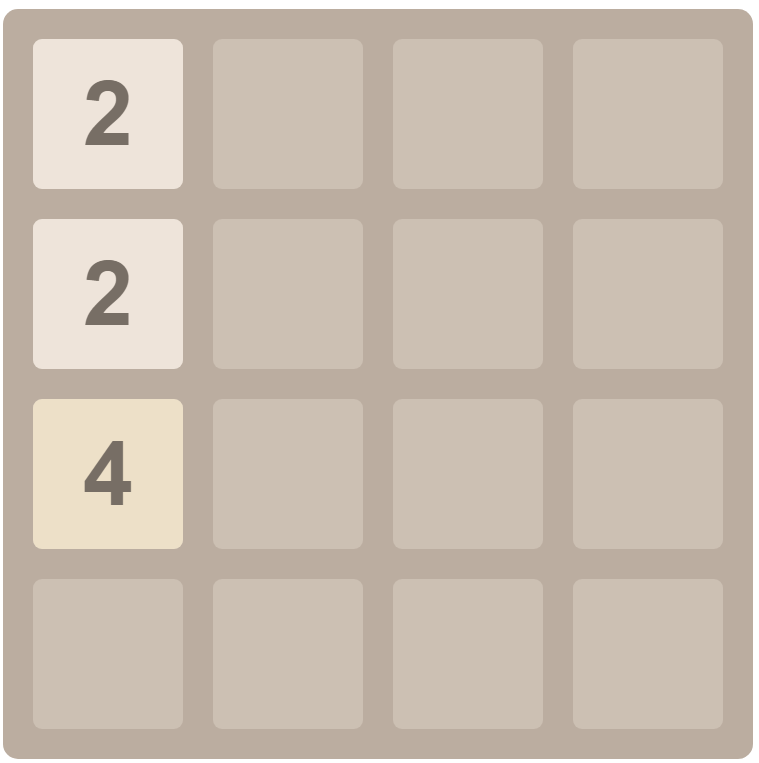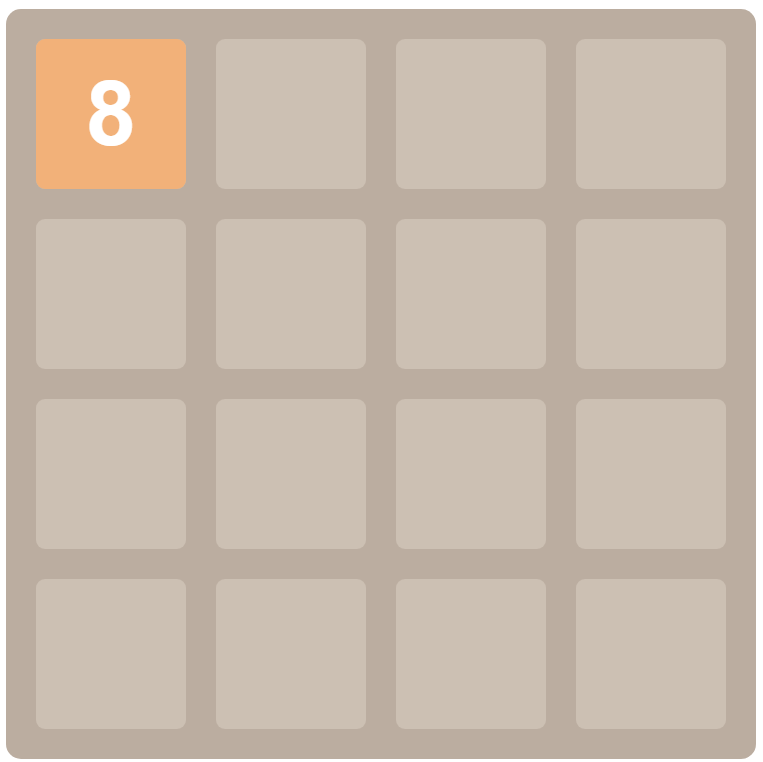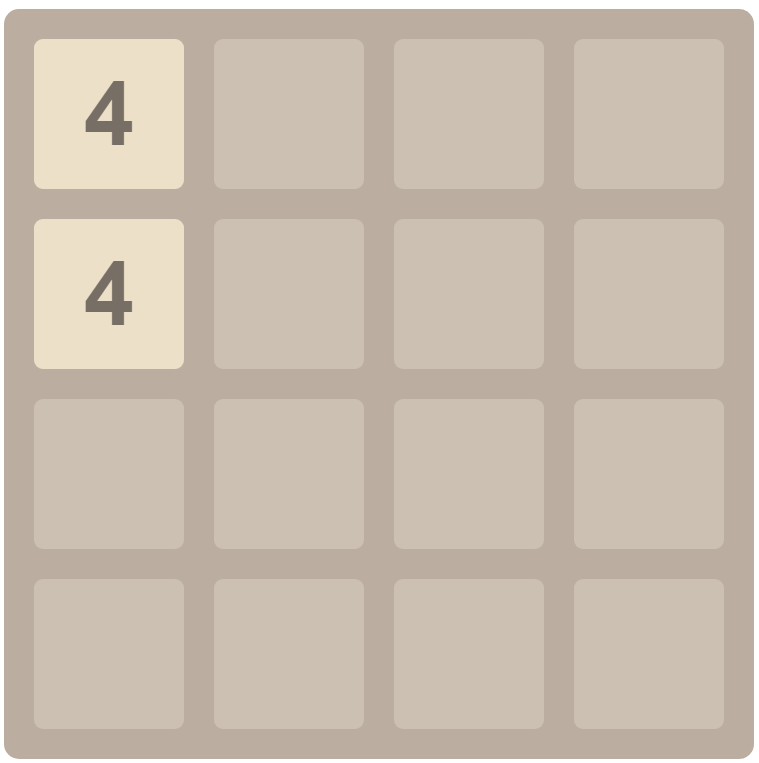/**
* 往上移动
*/
function moveUp() {
if (!canMoveUp()) {
// 如果不能移动
return false;
}

// 每一列上的方块可以移动到最顶端的那个行索引
let canMoveTopRowIndex = [0, 0, 0, 0];

for (let rowIndex = 1; rowIndex < 4; rowIndex++) {
for (let columnIndex = 0; columnIndex < 4; columnIndex++) {
if (chessBoard[rowIndex][columnIndex] !== 0) {
for (let k = canMoveTopRowIndex[columnIndex]; k < rowIndex; k++) {
if (
chessBoard[k][columnIndex] === 0 &&
noBlockVer(columnIndex, k, rowIndex)
) {
// 上侧为空，不存在障碍物
showMoveAnimation(rowIndex, columnIndex, k, columnIndex);
// 移动过去
chessBoard[k][columnIndex] = chessBoard[rowIndex][columnIndex];
// 之前的消失
chessBoard[rowIndex][columnIndex] = 0;
continue;
} else if (
chessBoard[k][columnIndex] === chessBoard[rowIndex][columnIndex] &&
noBlockVer(columnIndex, k, rowIndex)
) {
showMoveAnimation(rowIndex, columnIndex, k, columnIndex);
chessBoard[k][columnIndex] = 2 * chessBoard[rowIndex][columnIndex];
chessBoard[rowIndex][columnIndex] = 0;
score.value = score.value + chessBoard[k][columnIndex];
canMoveTopRowIndex[columnIndex] = k + 1;
continue;
}
}
}
}
}
return true;
}

1
2
3
4
5
6
7
8
9
10
11
12
13
14
15
16
17
18
19
20
21
22
23
24
25
26
27
28
29
30
31
32
33
34
35
36
37
38
39
40
41
42
43
44

#### # 3.3.3 游戏结束的判断

1. 方格上没有空白空间了
2. 方格上的所有点都不能移动了，上下左右四个方向都不能移动了。

/**
* 判断棋盘中还有空间吗
*/
function noSpace() {
for (let i = 0; i < 4; i++) {
for (let j = 0; j < 4; j++) {
// 还有空间
if (chessBoard[i][j] === 0) {
return false;
}
}
}
return true;
}

1
2
3
4
5
6
7
8
9
10
11
12
13
14

/**
* 判断是否可以移动
*
* @returns false 可以移动, true 无法移动
*/
function noMove() {
// 只要有一个方向可以移动，就能移动
if (canMoveDown() || canMoveLeft() || canMoveRight() || canMoveUp()) {
return false;
}

// 无法移动
return true;
}

1
2
3
4
5
6
7
8
9
10
11
12
13
14

## # 四、游戏结果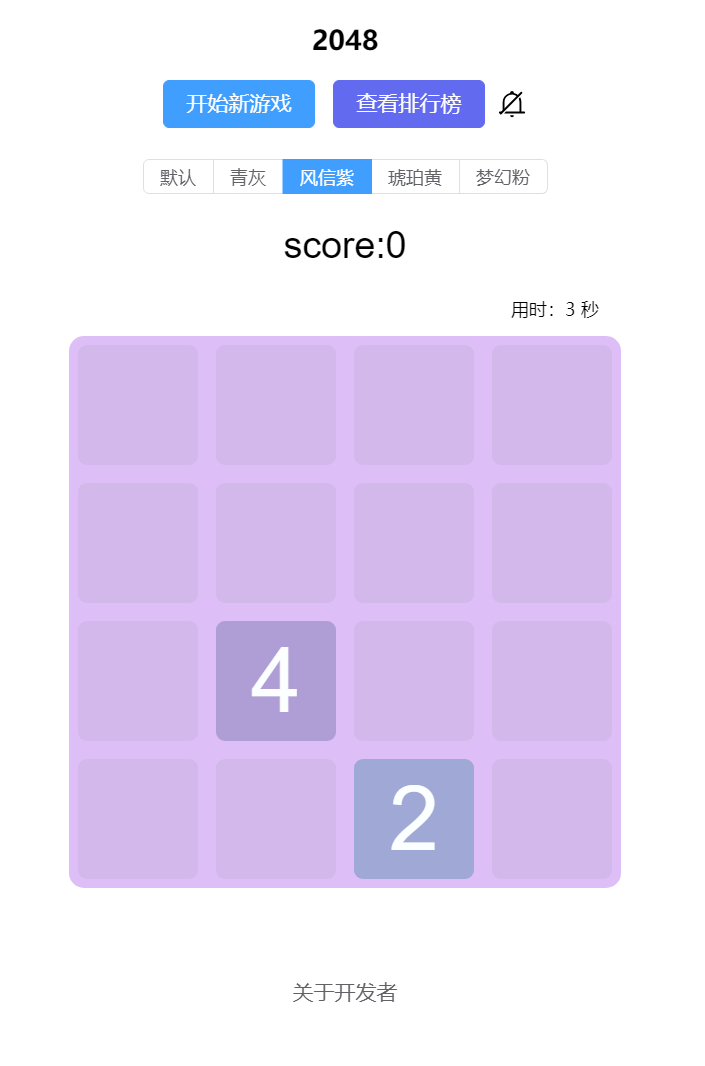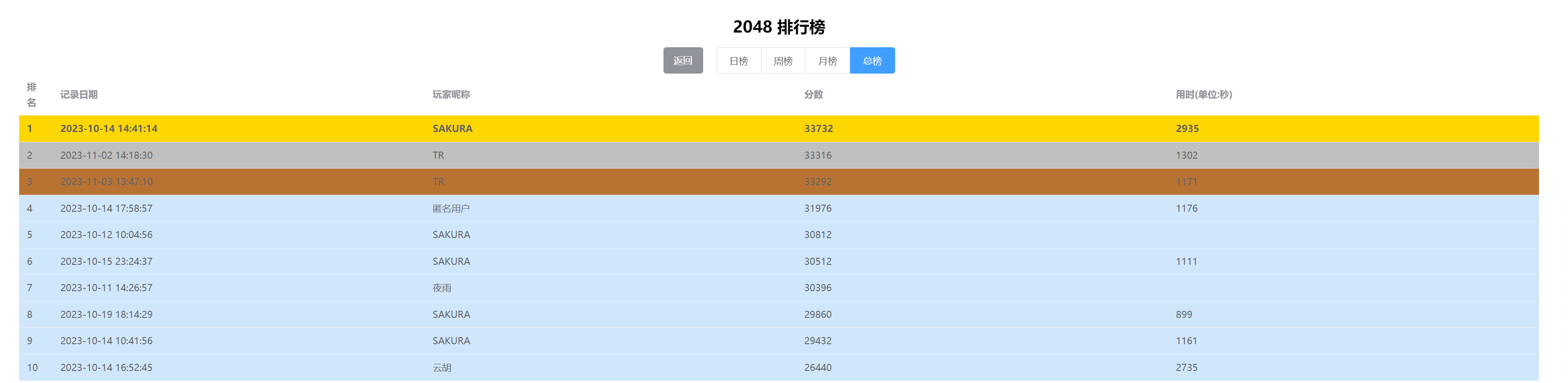GitHub 仓库地址：https://github.com/stevenling/vue-2048 (opens new window)